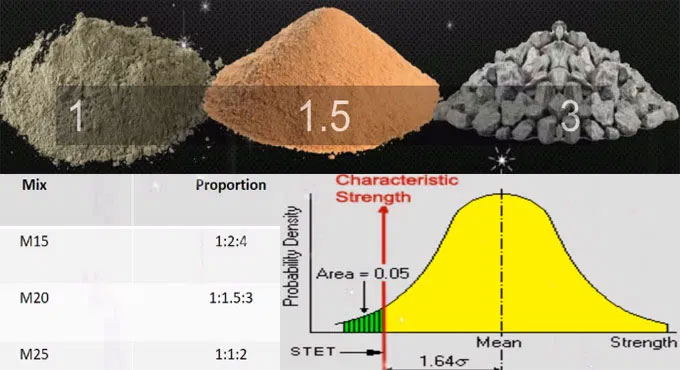# A discussion on the Quantity of Water Required for M20 Grade ConcreteYou will learn about the quantity of water that is required for M20 grade concrete. The concrete is made of cement, sand, aggregate and water. To acquire the required strength, it is extremely crucial to get the water-cement ratio right. For every different kind of concrete there is a different proportion. Different quantity of water is required with the different grade of concrete mix. Shrinkage and creep can occur if excessive amount of water is used.

M20 grade of concrete requires 200 liters or 53 gallons of water.

Given below is a list of water required for different kind of concretes:

The ?M? refers to the Mix and value behind M refers to the Compressive strength of concrete in KN/cu.mat 28 days.

For M5 mix, whose ratio is 1:5:10, the weight of cement (in kgs) is 141.00, the weight of sand in Kgs is 785.00, water in liters required is 70.50

For M7.5 mix, whose ratio is 1:4:8, the weight of cement is 174.00kg, the weight of sand is 773kgs, water required for it is 87liters.

For M10 mix, whose ratio is 1:3:6, the weight of cement is 226.00kg, the weight of sand is 753.60kgs, water required for it is 113 liters.

For M15 mix, whose ratio is 1:2:4, the weight of cement is 322kg, the weight of sand is 717.80kgs, water required for it is 161 liters.

For M20 mix, whose ratio is 1:1.5:3, the weight of cement is 403.20kg, the weight of sand is 672 kgs, water required for it is 201.60 liters.

For M25 mix, whose ratio is 1:1:2, the weight of cement is 565.00kgs, the weight of sand is 565.00kgs, water required for it is 282.50liters.

For M30 mix, whose ratio is 1:1:3, the weight of cement is 452 kgs, the weight of sand is 452.00kgs, water required for it is 226 liters.